``````struct TreeNode {
int val;
TreeNode *left;
TreeNode *right;
};

void traversal(TreeNode *root) {
if (root == nullptr)
return ;

// 前序遍历
traversal(root->left);
// 中序遍历
traversal(root->right);
// 后序遍历
}
``````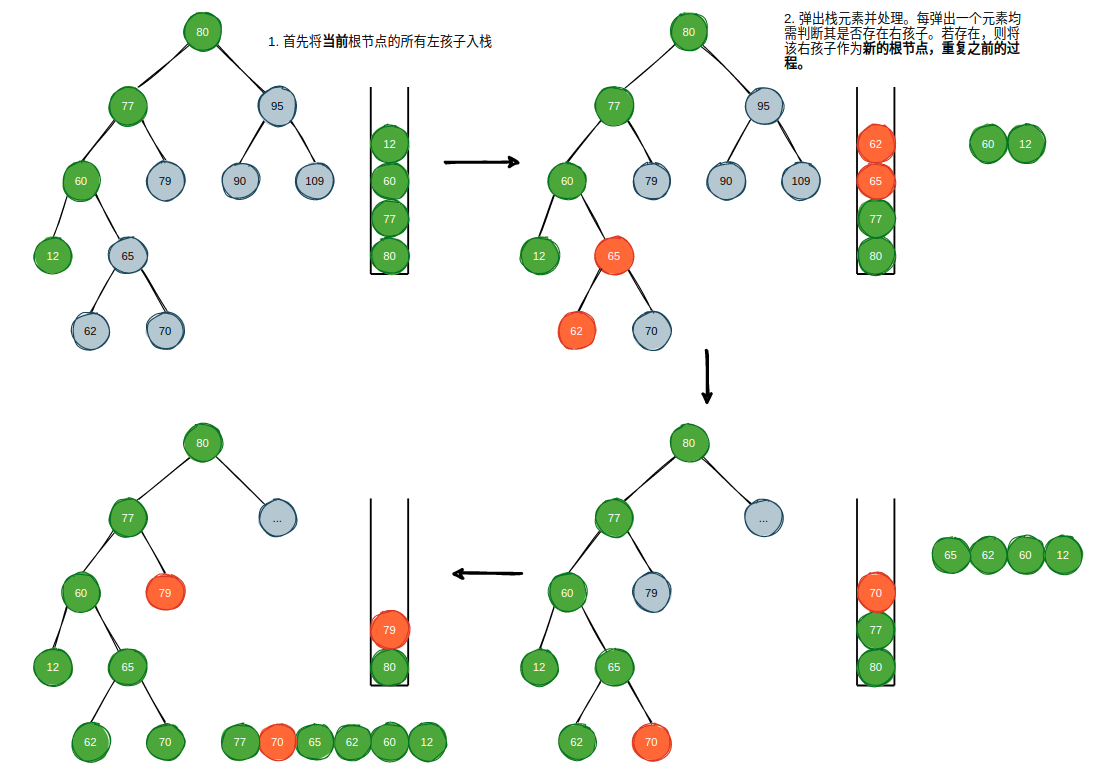``````vector<int> inorderTraversal(TreeNode* root) {

vector<int> result;
stack<TreeNode *> depthStack;

if (root == nullptr) return result;

TreeNode *curRoot = root;
depthStack.push(curRoot);

while (!depthStack.empty()) {

while (curRoot->left != nullptr) {
depthStack.push(curRoot->left);
curRoot = curRoot->left;
}

TreeNode *current = depthStack.top();
result.push_back(current->val);
depthStack.pop();

if (current->right != nullptr) {
depthStack.push(current->right);
curRoot = current->right;
}
}
return result;
}
``````

``````int climbStairs(int n) {
if (n <= 2)
return n;
return climbStairs(n-1) + climbStairs(n-2);
}
``````

``````int climbStairs(int n) {
if (n <= 2)
return n;
int left = climbStairs(n-1);
int right = climbStairs(n-2);
return left + right;
}
``````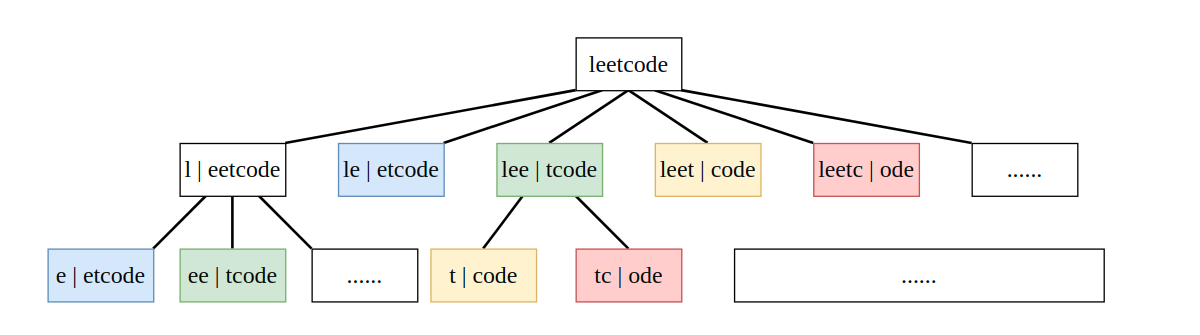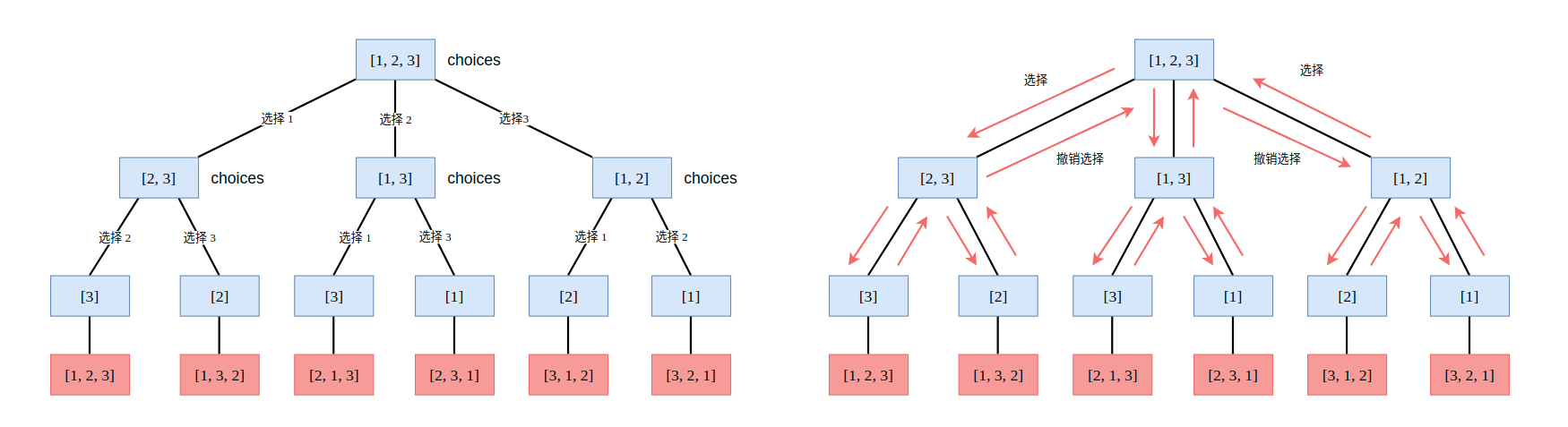### 1. 简单地二叉树遍历问题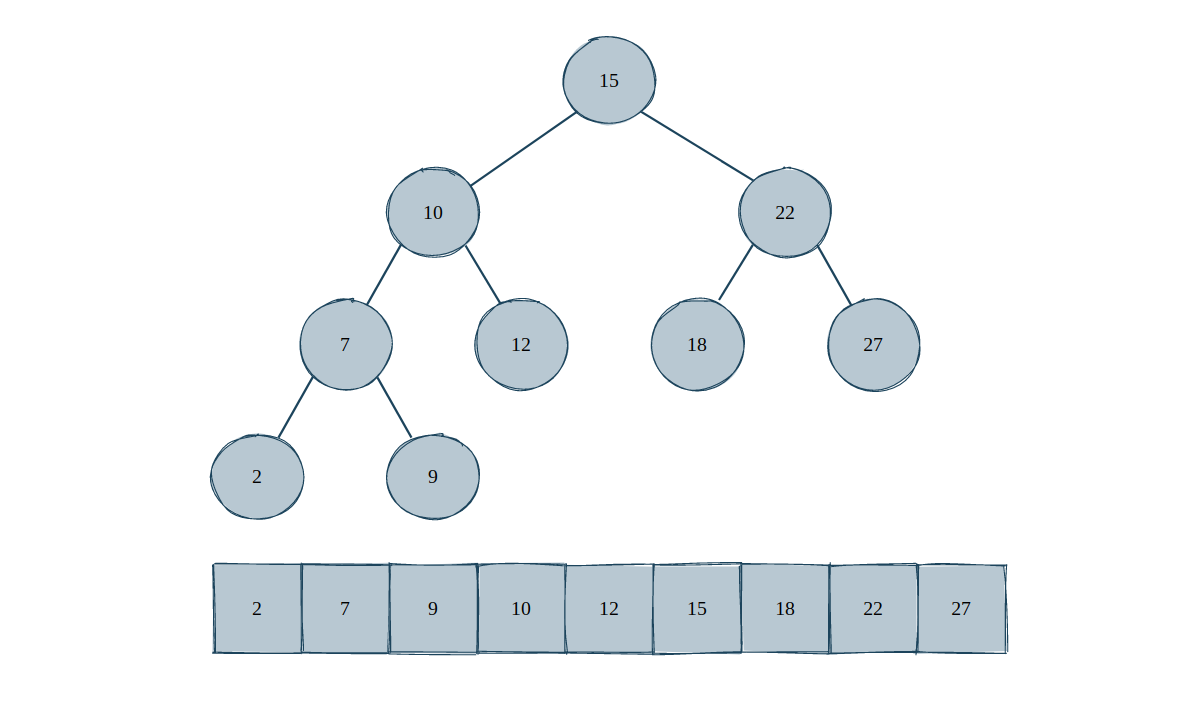``````class Solution {
private:
vector<int> result;
void inorder(TreeNode* root, int k) {
if (root == NULL) return;

inorder(root->left, k);

// 中序遍历访问节点
result.push_back(root->val);
if (result.size() == k) return;

inorder(root->right, k);
}
public:
int kthSmallest(TreeNode* root, int k) {
midorder_dfs(root, k);
return result[k-1];
}
};
``````

226. Invert Binary Tree，反转一棵二叉树，使得所有节点的左子节点变成右子节点，右子节点变成左子节点。据说是 Max Howell 未能加入 Google 的直接原因，不过这我是不相信的，个人更倾向于认为是 Max Howell 和 Google 在面试的内容产生了分歧: 反转二叉树有个锤子用? 能反转二叉树的人就能写出 Homebrew 了?

``````struct TreeNode* invertTree(struct TreeNode* root){

if (root == NULL) return NULL;

root->left = invertTree(root->left);
root->right = invertTree(root->right);

struct TreeNode *temp = root->left;
root->left = root->right;
root->right = temp;

return root;
}
``````

114. Flatten Binary Tree to Linked List，题目要求将一棵二叉树展开成一个链表形式的树，如下所示:

``````Input:
1
/ \
2   5
/ \   \
3   4   6

Output:
1
\
2
\
3
\
4
\
5
\
6
``````

``````void flatten(TreeNode* root) {
if (root == nullptr) return;

if (root->left != nullptr)
flatten(root->left);

if (root->right != nullptr)
flatten(root->right);

TreeNode *temp = root->right;

root->right = root->left;
root->left = nullptr;

// 找到新的右子节点的最后一个右子节点
while (root->right != nullptr)
root = root->right;

root->right = temp;
}
``````

### 2. 通过 DFS 结果恢复二叉树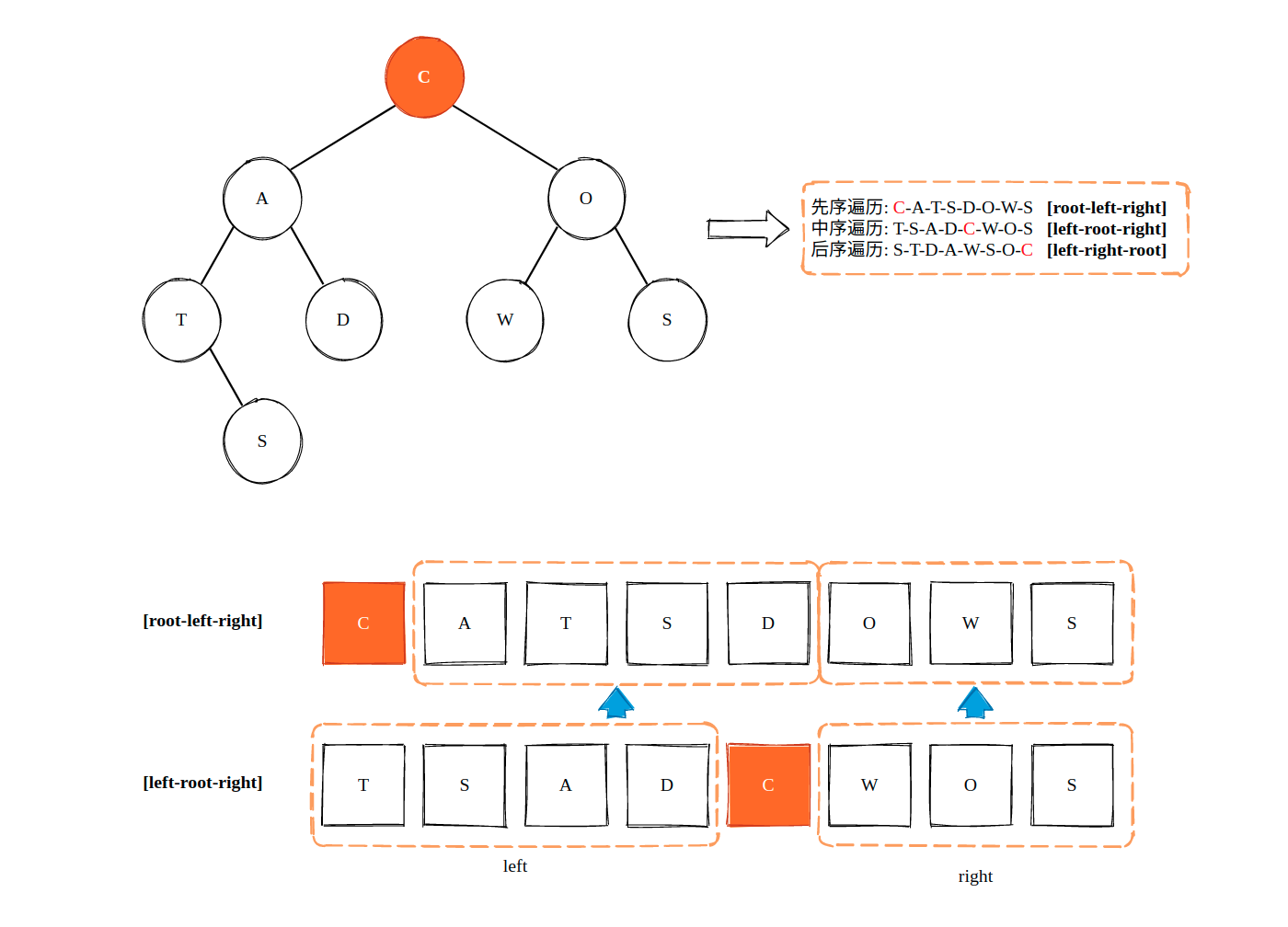``````class Solution {
private:
TreeNode *traverse(vector<int>& preorder, int preLeft, int preRight, vector<int>& inorder, int inLeft, int inRight, unordered_map<int, int> &inHashmap) {

if (preLeft > preRight || inLeft > inRight)
return nullptr;

TreeNode *root = new TreeNode(preorder[preLeft]);

int inRootIndex = inHashmap[root->val];
int leftNums = inRootIndex - inLeft;

root->left = traverse(preorder, preLeft + 1, preLeft + leftNums, inorder, inLeft, inRootIndex - 1, inHashmap);
root->right = traverse(preorder, preLeft + leftNums + 1, preRight, inorder, inRootIndex + 1, inRight, inHashmap);

return root;
}
public:
TreeNode* buildTree(vector<int>& preorder, vector<int>& inorder) {

unordered_map<int, int> inHashmap;

for (int i = 0; i < inorder.size(); i++)
inHashmap[inorder[i]] = i;

return traverse(preorder, 0, preorder.size() - 1, inorder, 0, inorder.size() - 1, inHashmap);
}
};
``````

### 4. 小结

``````void traversal(TreeNode *root) {
if (root == nullptr) return ;
// 前序遍历
traversal(root->left);
// 中序遍历
traversal(root->right);
// 后序遍历
}
``````

``````void traversal(TreeNode *root) {
for (auto child: TreeNode->childs)
traversal(child);
}
``````

leetcode 关于二叉树这一话题的全部题目:

https://leetcode.com/tag/tree/

https://github.com/SmartKeyerror/Snorlax/tree/master/leetcode/tree https://github.com/SmartKeyerror/Snorlax/tree/master/leetcode/binary-search-tree